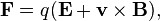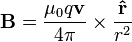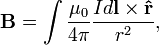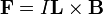# Electromagnetic field energy

paweld
I wonder why we always assume that electromagnetic field energy density is
$$w = \frac{1}{2}(E^2+B^2)$$. In fact energy of the filed can be any
function $$w$$ for which there exists such vectror field $$\vec{S}$$ that
continuity equation is fulfilled:
$$\vec{E} \vec{J}+\frac{\partial w}{\partial t}+\nabla \vec{S}=0$$.
Where $$\vec{E} \vec{J}$$ is a work donw by the filed in unit volume and time.
Obviously we can choose: $$w = \frac{1}{2}(E^2+B^2)$$ and
$$S =\vec{E}\times \vec{B}$$ but there are also other options.
Because $$\frac{\partial \rho}{\partial t}+\nabla \vec{j}=0$$ ($$\rho$$ - density
of charge, $$\vec{j}$$ - density of current) we can take for example:
$$w = \frac{1}{2}(E^2+B^2) + \alpha \rho$$ and $$S =\vec{E}\times \vec{B} + \alpha\vec{j}$$.
This change doesn't affect the continuity equation and of course both $$\rho$$ and $$\vec{j}$$ can by express
by means of Maxwell equation in terms of $$\vec{E}$$ and $$\vec{B}$$
so that $$w$$ and $$\vec{S}$$ depend only on the field.
Are there any physical arguments for $$w = \frac{1}{2}(E^2+B^2)$$ or it's
only our traditional choice and others are as well.

alan.b
Magnitude of magnetic field B is proportional to the velocity and have geometrical relations with this vector that E does not have, therefore my conclusion is that your equation is indeed wrong, and having E and B forces acting in different (perpendicular) directions might be serious problem to calculate 'work done'. Also, I could not find that formula in Wikipedia, but I suppose some article there must say something more about the origin of that equation.

jackiefrost
These stem from the separate derivations of W for electric field and magnetic fields. W for electric field results from integrating the product of electric potential and charge density over all space and W for magnetic field from integrating the scalar product of magnetic vector potential and current density vector. As a result of these integrations, W for electric field calculates to e0 E2/2 per unit volume and W for magnetic field is B2/2u0 per unit volume.

paweld
Yes, you are right jackiefrost. I didn't take into account that we know the energy of
static electric and magnetic energy density and these formula should be consistent.
This removes the arbitrariness in choice of w.
But I think that there should exist also some relativistic arguments for this particular choice.

alan.b
Hold on a second.There is this velocity vector having very intimate relation with the magnetic field. Both, magnetic field and magnetic force magnitude are proportional, and perpendicular, to the velocity vector, so where is this 'v' in that formula, where did it go?

W for magnetic field from integrating the scalar product of magnetic vector potential and current density vector.

That can mean only one thing - use Biot-Savart law to calculate magnetic field potential and Lorentz force equation to calculate magnetic force (on a "test particle"), except that you seem to be talking about "current in a wire", so the 'velocity' should be integrated via amperes, like this:In any case, the equation in the opening post can not be valid, there is no way that can be derived without keeping the relation between magnetic field, as well as magnetic force, with the velocity vector. Also, how can scalar help you with calculating work done, especially if displacement was in perpendicular direction to the flow of the current?

Last edited by a moderator:
Gold Member
The equation given by the OP are indeed correct. I'm not sure what you are trying to do here but it isn't correct. This expression is for the energy density of the electric and magnetic fields, charges do not come into this. If you wanted the energy density of sources they would be something like the product of the potentials and currents.

Second, the Biot-Savart law is not correct except in the quasi-static limit. Third, the Biot-Savart law does not calculate the magnetic vector potential.

The energy density of the field is basic introductory electromagnetics.

alan.b
Energy density is nothing more but 'potential energy' aka field potential like with gravity, only per volume area instead of per point particle, it is "approximated" with Biot-Savart in either case.

Of course charges come into this, where else would you get electric fields from, and if those charges did not move, where else would you get magnetic fields from?

If not Biot-Savart, then what equation do you suggest was used to derive that formula, is there any such equation for magnetic field magnitude that is not related to charge velocity?

So, what I'm trying to do is to point out that energy density of the magnetic field is related to the velocity and this relation is missing from that equation. In other words, I do not think you, or anyone, can derive it and cancel out velocity variable, nor can energy density of magnetic field be calculated without Biot-Savart law. Just try and calculate energy density of a single electron's magnetic field and see what equations will you end up.